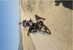# New to Qlik Sense

If you’re new to Qlik Sense, start with this Discussion Board and get up-to-speed quickly.

Announcements
Don't miss the upcoming Q&A with Qlik session on Qlik Application Automation on November 16th! REGISTER NOW
cancel
Showing results for
Did you mean:Creator

## the use of "*/" in set analysis

Can anyone tell me what is the meaning of  "*/"   in this set analysis please {<date={"*/\$(=Year(Max(date)-1))"}   ?

Labels (1)
• ### Other

1 Solution

Accepted SolutionsSpecialist II

Not sure they can be used together since * means Intersection and / means Symmetric Difference.

{<date={"*\$(=Year(Max(date)-1))"}>}  this returns all "date" where the year is equal to the year of Max(date)-1 according to current selections.

{<date={"/\$(=Year(Max(date)-1))"}>}  this returns all "date" where the year is not equal to the year of Max(date)-1 and not equal to current selections

3 RepliesSpecialist II

Here you can find the information you need:Creator
Author

Thank you @micheledenardi  for the reply, i have read the article, it's interesting, however i can't understand the meaning of this formula:

Max(TOTAL {<date={"*/\$(=Year(Max(date)-1))"}>} Aggr(If(date=Max(TOTAL <id_invested> date,2), Max(position)),id_invested,date))

can you help by interpreting the meaning of   {<date={"*/\$(=Year(Max(date)-1))"}>} here please?

Thank you.Specialist II

Not sure they can be used together since * means Intersection and / means Symmetric Difference.

{<date={"*\$(=Year(Max(date)-1))"}>}  this returns all "date" where the year is equal to the year of Max(date)-1 according to current selections.

{<date={"/\$(=Year(Max(date)-1))"}>}  this returns all "date" where the year is not equal to the year of Max(date)-1 and not equal to current selectionsTags
Community Browser